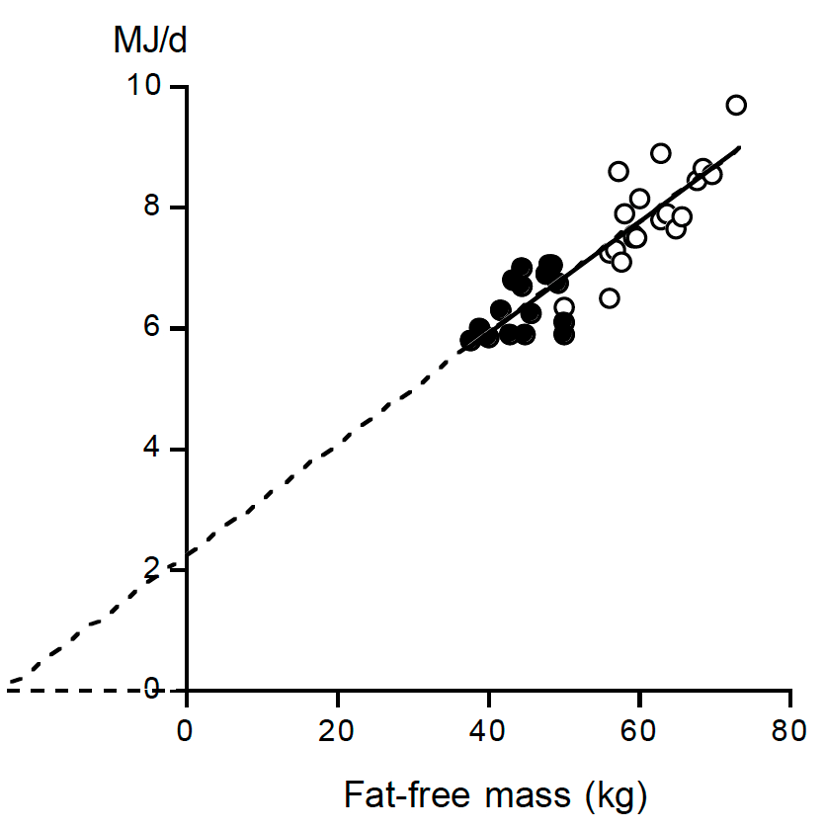# etx-obesity-ch7-fig3Figure 3. Resting energy expenditure (REE) plotted as a function of fat-free mass for the subjects from reference 5 as described in Figure (2) (17 women: closed symbols; 20 men: open symbols) with the calculated linear regression line (REE (MJ/d) = 2.27 + 0.091 fat-free mass (kg), r2 = 0.78).